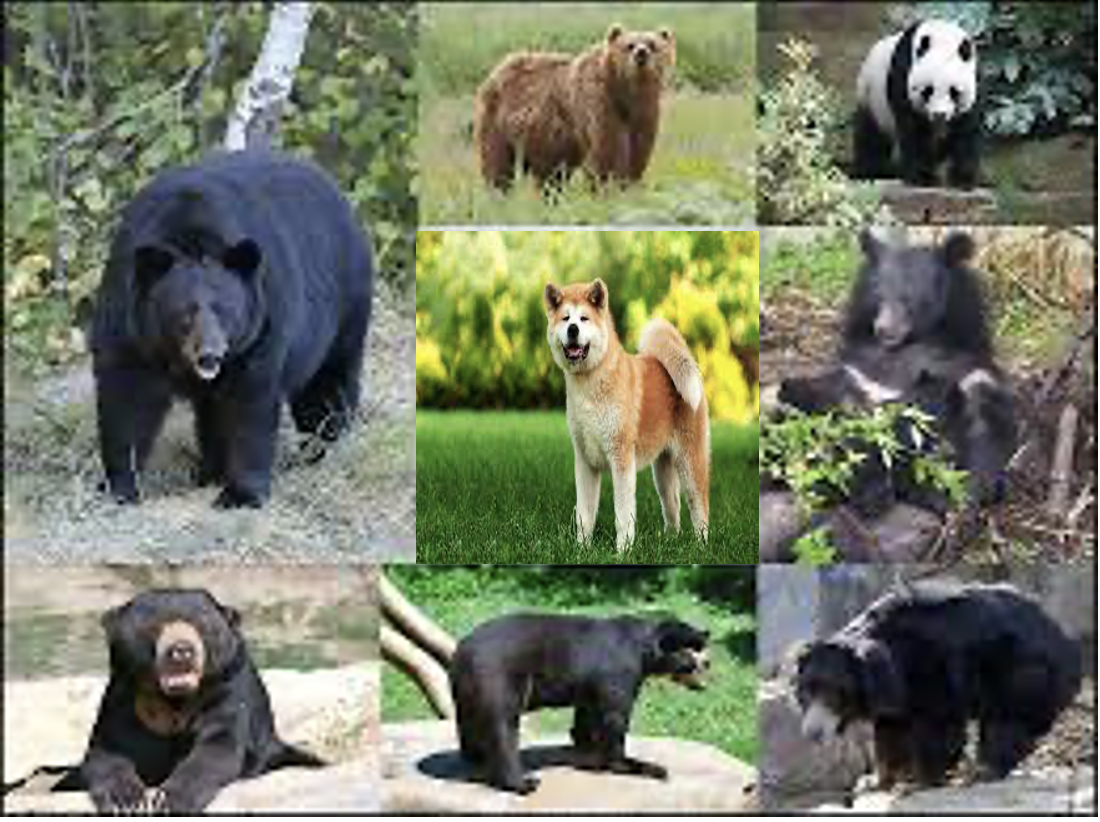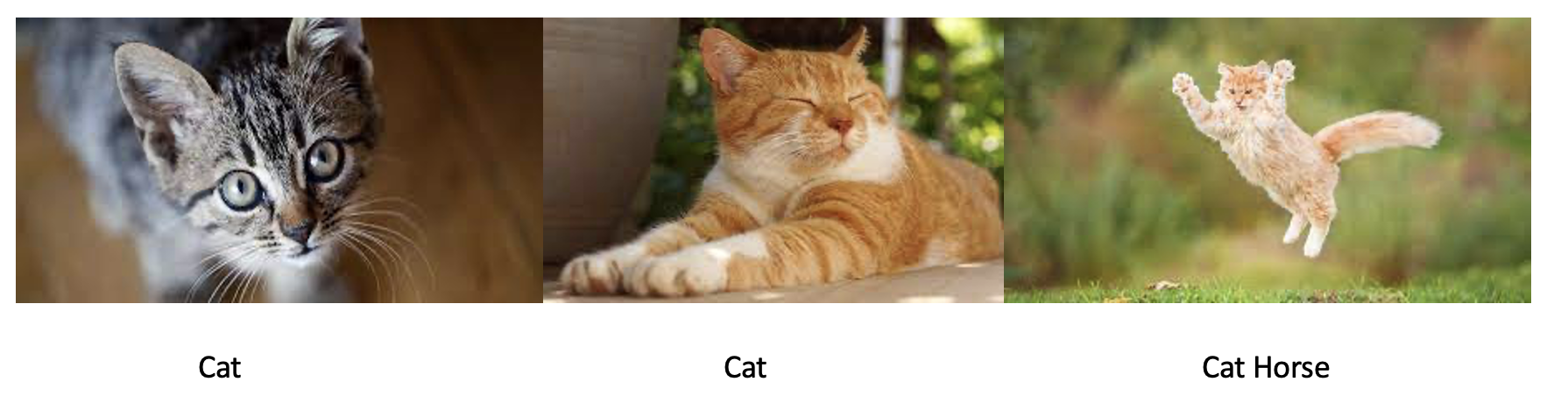### Keypoints#

#### Multi-label classification#

• Multi-label classification refers to the problem of identifying the categories of objects in images that may not contain exactly one type of object.
• There may be more than one kind of object, or there may be no objects at all in the classes that you are looking for.
• See two examples below where we have a bear dataset with a dog included named bear and another example where the cat is classified as cat and horse.• As a note, in FastAI we can handle the multi-labels with MultiCategoryBlock which will encode all the vocabulary into a list of 0’s and have 1s where data is present.
• So, by checking where 1’s are we can identify which category(s) that image belongs to.
• This technique of representing the data in 1’s on a vector of 0s is called One-hot encoding.

#### Binary Cross-Entropy Loss#

• In the case of single category labels, we have the cross-entropy loss.
• The Cross-Entropy loss is a combination of using the negative log likelihood on the log values of the probabilities from the softmax function.
• But in the case of multi-category labels, we don’t have the probabilities rather we have the one-hot encoded values.
• In this case, the best option would be the binary cross-entropy loss which is basically just mnist_loss along with log.
 ``````1 2 3 `````` ``````def binary_cross_entropy(inputs, targets): inputs = inputs.sigmoid() return -torch.where(targets==1, 1-inputs, inputs).log().mean() ``````
• So, once we are ready with the data and preparing to create Learner for training, we do not need to explicitly provide the loss. The FastAI will pick up the binary corss-entorpy loss by default.
• Now that we have the loss ready, we need to pick a metric which is accuracy by default for all the classification problems we worked on.
• But the accuracy is not a good fit for this problem of multi-label since for each image we could have more than one prediction. So we need to use accuracy_multi with a threshold that will address the problem.

Note: Since the threshold for the accuracy_multi is by default 0.5, we can override the function using the partial function from python.

Example of the Learner in this case:

 ``````1 2 `````` ``````learn = cnn_learner(dls, resnet50, metrics=partial(accuracy_multi, thresh=0.2)) learn.fine_tune(3, base_lr=3e-3, freeze_epochs=4) ``````

#### Regression#

• A regression problem is when the output variable is a real or continuous value, such as “salary” or “weight”.
• Many different models can be used, the simplest is the linear regression. It tries to fit data with the best hyper-plane which goes through the points.• An image regression problem refers to learning from a dataset where the independent variable is an image, and the dependent variable is one or more floats.
• And image regression is simply a CNN under the hood. One of the key perspective to consider while building a datablock for regression is to use pointblock instead of a category block since the labels represents coordinates.
• Another important point to remember while construction the Learner is to provide the y_range=(-1,1) attribute to make sure that we give the range of the rescaled coordinates.
 ``````1 `````` ``````learn = cnn_learner(dls, resnet18, y_range=(-1,1)) ``````
• In the case of the regression problem, the loss that can used is MSELoss (Mean Squared Error loss).
• The MSE tells you how close a regression line is to a set of points. It does this by taking the distances from the points to the regression line (these distances are the “errors”) and squaring them.

#### Conclusion#

• All the problems like single-label classification, multi-label classification & regression seems to work on basis of same model except for the loss function that changes every time.
• So, we need to keep an eye on hyper parameters and loss which will effect the results.